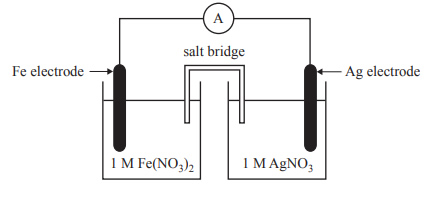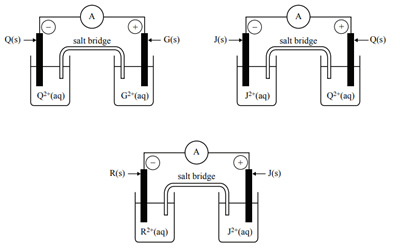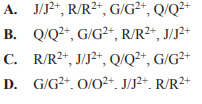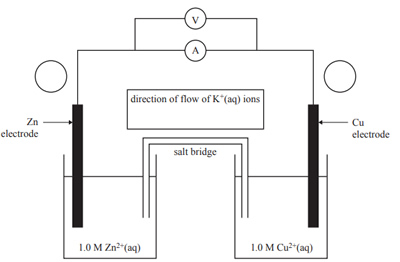Electrochemical (2018 VCE) 1) Which one of the following statements is the most accurate? A. All fuel cells are galvanic cells. B. All galvanic cells are primary cells. C. All secondary cells have porous electrodes. D. All fuel cells are more efficient than all secondary cells. Solution2) A galvanic cell is set up as shown in the diagram belowWhen this cell is operating A. a gas forms at the Ag electrode. B. the mass of the Ag electrode increases. C. Ag+ ions move towards the Fe electrode. D. electrons move from the Ag electrode to the Fe electrode. Solution3) The silver oxide-zinc battery is rechargeable and utilises sodium hydroxide, NaOH, solution as the electrolyte. The battery is used as a backup in spacecraft, if the primary energy supply fails. The overall reaction during discharge is Zn + Ag2O → ZnO + 2Ag When the silver oxide-zinc battery is being recharged, the reaction at the anode is A. 2Ag + 2OH– → Ag2O + H2O + 2e– B. Ag2O + H2O + 2e– → 2Ag + 2OH– C. ZnO + H2O + 2e– → Zn + 2OH– D. Zn + 2OH– → ZnO + H2O + 2e– Solution4) The following diagrams represent combinations of four galvanic half-cells (G/G2+, J/J2+, Q/Q2+ and R/R2+) that were investigated under standard conditions. Each half-cell consisted of a metal electrode placed in a 1.0 M nitrate solution of the respective metal ion. The diagrams show the polarity of the electrodes in each half-cell, as determined using an ammeter. The results were then used to determine the order of the E0 values of the half-reactions.Which of the following indicates the order of the half-cell reactions, from the lowest E0 value to the highest?Solution5) Redox reactions occur in the human body as well as in electrochemical cells. a. Nicotinamide adenine dinucleotide (NAD) is a vital coenzyme for energy production in the human body. It exists in two forms: an oxidised form, NAD+, and a reduced form, NADH. NAD is involved in the conversion of ethanol, CH3CH2OH, to ethanal, H3CHO, in the human body. The overall equation for this redox reaction is CH3CH2OH + NAD+ → CH3CHO + NADH + H+ i. Write the two half-equations for this redox reaction. States are not required. Oxidation half-equation Reduction half-equation Solution ii. Identify the reducing agent in this redox reaction. Solution6) The Daniell cell, a type of galvanic cell, was first constructed in the mid-1800s and this type of cell is still in use today. A diagram of the Daniell cell is shown belowi. Label the polarity of the electrodes by placing a positive (+) or negative (–) sign in each of the circles next to the electrodes on the diagram above. Solution ii. Use the electrochemical series to determine the theoretical voltage of this cell. Solution iii. The electrolyte in the salt bridge is a potassium nitrate solution, KNO3(aq). In the box above the salt bridge, use an arrow to indicate the direction of flow of K+(aq) ions. Solution iv. List two visible changes that are likely to be observed when the Daniell cell has been operating for some time. Solution v. What design features of the Daniell cell structure would allow it to produce electrical energy? Solution